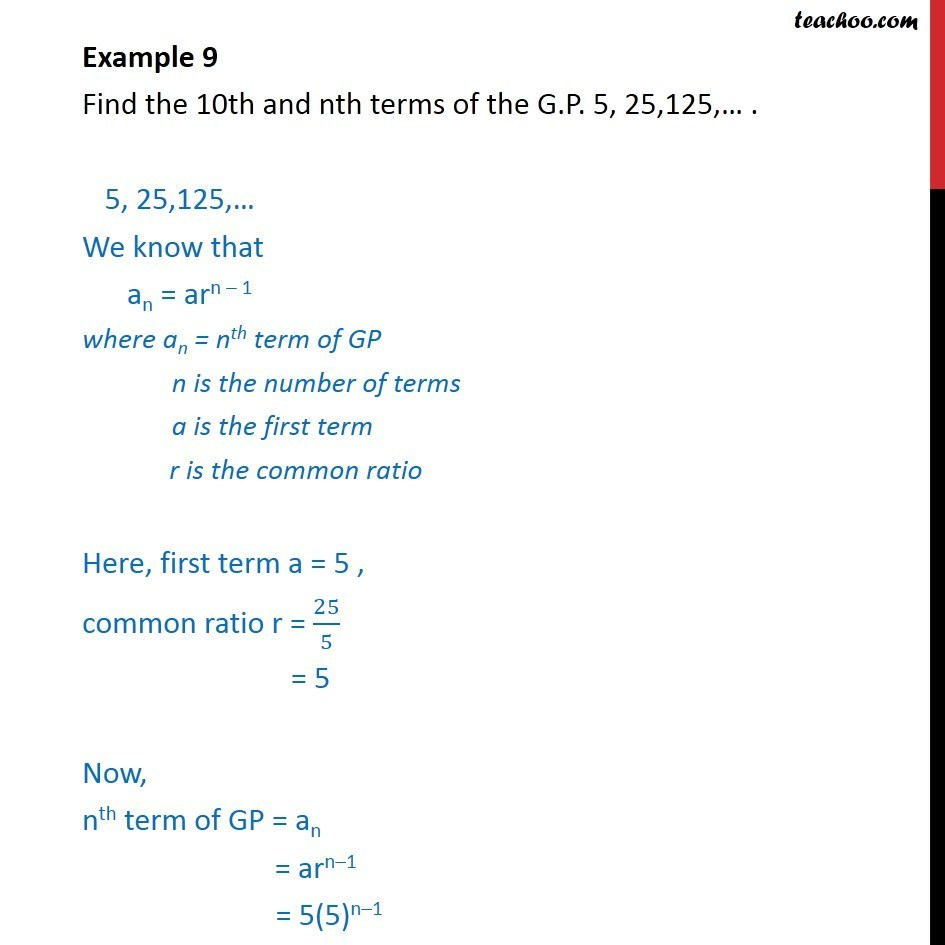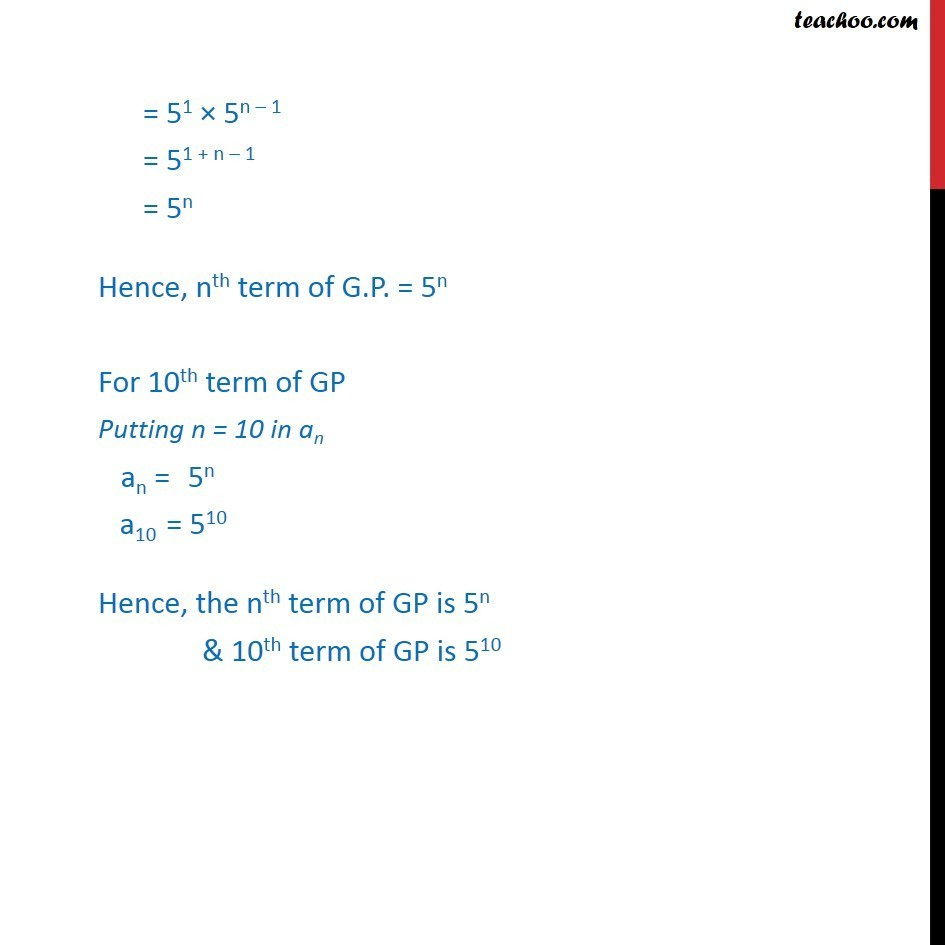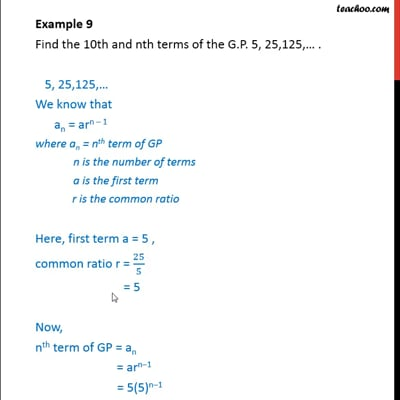Examples

Chapter 9 Class 11 Sequences and Series (Term 1)
Serial order wiseThis video is only available for Teachoo black users

### Transcript

Example 9 Find the 10th and nth terms of the G.P. 5, 25,125, . 5, 25,125, We know that an = arn 1 where an = nth term of GP n is the number of terms a is the first term r is the common ratio Here, first term a = 5 , common ratio r = 25/5 = 5 Now, nth term of GP = an = arn 1 = 5(5)n 1 = 51 5n 1 = 51 + n 1 = 5n Hence, nth term of G.P. = 5n For 10th term of GP Putting n = 10 in an an = 5n a10 = 510 Hence, the nth term of GP is 5n & 10th term of GP is 510